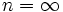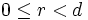# Dihedral group

WARNING: POTENTIAL TERMINOLOGICAL CONFUSION: Please don't confuse this with dicyclic group (also called binary dihedral group)
This article defines a group property: a property that can be evaluated to true/false for any given group, invariant under isomorphism
View a complete list of group properties
VIEW RELATED: Group property implications | Group property non-implications |Group metaproperty satisfactions | Group metaproperty dissatisfactions | Group property satisfactions | Group property dissatisfactions

This is a family of groups parametrized by the natural numbers, viz, for each natural number, there is a unique group (upto isomorphism) in the family corresponding to the natural number. The natural number is termed the parameter for the group family

## Definition

The dihedral group of degree$n$ and order$2n$, denoted sometimes as$D_n$, sometimes as$\operatorname{Dih}_n$, and sometimes as$D_{2n}$ (this wiki uses$D_{2n}$) is defined in the following equivalent ways:$\langle x,a \mid a^n = x^2 = e, xax^{-1} = a^{-1} \rangle$

• (For$n \ge 3$): It is the group of symmetries of a regular$n$-gon in the plane, viz., the plane isometries that preserves the set of points of the regular$n$-gon.

The dihedral groups arise as a special case of a family of groups called von Dyck groups. They also arise as a special case of a family of groups called Coxeter groups.

Note that for$n = 1$ and$n = 2$, the geometric description of the dihedral group does not make sense. In these cases, we use the algebraic description.

The infinite dihedral group, which is the$n = \infty$ case of the dihedral group and is denoted$D_\infty$ and is defined as:$\langle x,a \mid x^2 = e, xax^{-1} = a^{-1} \rangle$.

## Particular cases

### For small values

Note that all dihedral groups are metacyclic and hence supersolvable. A dihedral group is nilpotent if and only if it is of order$2^k$ for some$k$. It is abelian only if it has order$2$ or$4$.

Order of group Degree (size of regular polygon it acts on) Common name for the group Comment
2 1 Cyclic group:Z2 Not usually considered a dihedral group.
4 2 Klein four-group elementary abelian group that is not cyclic
6 3 symmetric group:S3 metacyclic, hence supersolvable but not nilpotent
8 4 dihedral group:D8 nilpotent but not abelian
10 5 dihedral group:D10 metacyclic, hence supersolvable but not nilpotent
12 6 dihedral group:D12 direct product of the dihedral group of order six and the cyclic group of order two.
16 8 dihedral group:D16 nilpotent but not abelian

## Arithmetic functions$n$ here denotes the degree, or half the order, of the dihedral group, which we denote as$D_{2n}$.

Function Value Explanation
order$2n$ Cyclic subgroup of order$n$, quotient of order$2$.
exponent$\operatorname{lcm}(n,2)$ Exponent of cyclic subgroup is$n$, elements outside have order$2$.
derived length$2$ for$n \ge 3$,$1$ for$n = 1,2$
nilpotency class$k$ when$n = 2^k$, none otherwise Nilpotent only if$n$ is a power of$2$.
max-length 1 + sum of exponents of prime divisors of$n$ The dihedral groups are solvable.
composition length 1 + sum of exponents of prime divisors of$n$ The dihedral groups are solvable.
number of subgroups$\sigma(n) + d(n)$$\sigma(n)$ is the divisor sum function and$d(n)$ is the divisor count function.
number of conjugacy classes$(n+3)/2$ if$n$ is odd,$(n+6)/2$ if$n$ is even.
number of conjugacy classes of subgroups$3d(n) - d(m)$ where$m$ is the largest odd divisor of$n$$d(n)$ subgroups inside the cyclic part. For odd divisors, one external conjugacy class of subgroups per divisor; for even divisors, one external conjugacy class per divisor.

## Group properties

Property Satisfied Explanation
Abelian group False for$n \ge 3$ For$n \ge 3$, the elements$a,x$ do not commute.
Nilpotent group True for$n$ a power of$2$, false otherwise
Metacyclic group True
Supersolvable group True
Solvable group True
T-group True for$n$ odd or twice an odd number, false for$n$ a multiple of$4$
Rational group True for$n \le 4$, false for$n \ge 5$
Rational-representation group True for$n \le 4$, false for$n \ge 5$
Ambivalent group True Elements in the cyclic subgroup are conjugate via$x$, elements outside have order two.

## Subgroups

Further information: Subgroup structure of dihedral groups

There are two kinds of subgroups:

• Subgroups of the form$\langle a^d \rangle$, where$d|n$. The number of such subgroups equals the number of positive divisors of$n$, sometimes denoted$\tau(n)$. The subgroup generated by$a^d$ is a cyclic group of order$n/d$.
• Subgroups of the form$\langle a^d, a^r x \rangle$, where$d|n$ and$0 \le r < d$. The number of such subgroups equals the sum of all positive divisors of$n$, sometimes denoted$\sigma(n)$. The subgroup of the above form is a dihedral group of order$2n/d$.

In particular, all subgroups of the dihedral group are either cyclic or dihedral.

Also note that the dihedral group has subgroups of all orders dividing its order. This is true more generally for all finite supersolvable groups. Further information: Finite supersolvable implies subgroups of all orders dividing the group order

## Supergroups

### Groups having the dihedral group as quotient

The dicyclic group, also called the binary dihedral group, of order$4n$, has the dihedral group of order$2n$ as a quotient -- in fact the quotient by its center, which is a cyclic subgroup of order two. the presentation for the dicyclic group is given by:$\langle a,x \mid a^n = x^2 = (ax)^2 \rangle$.

Dicyclic groups whose order is a power of$2$ are termed generalized quaternion groups.